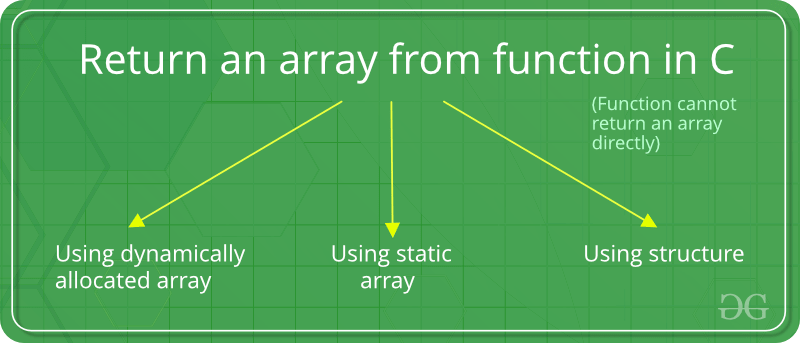# C语言函数如何返回数组？

8.8k

``````#include <stdio.h>

<strong>int</strong>* fun()
{
<strong>int </strong>arr;

/* 操作 arr[] */
arr = 10;
arr = 20;

<strong>return </strong>arr;
}

<strong>int </strong>main()
{
<strong>int</strong>* ptr = fun();
printf(%d %d, ptr, ptr);
<strong>return </strong>0;
} ``````

``````In function 'int* fun()':
int arr;
^``````

``10 20``## 方法一 函数外初始化数组

``````#include <stdio.h>

<strong>int</strong>* fun(<strong>int </strong>*arr)
{
/* 操作 arr[] */
arr = 10;
arr = 20;

<strong>return </strong>arr;
}

<strong>int </strong>main()
{
<strong>int </strong>arr;
<strong>int</strong>* ptr = fun(arr);
printf(%d %d, ptr, ptr);
<strong>return </strong>0;
} ``````

``10 20``

## 方法二 使用static数组

``````#include <stdio.h>

<strong>int</strong>* fun()
{
<strong>static int </strong>arr;

/* 操作 arr[] */
arr = 10;
arr = 20;

<strong>return </strong>arr;
}

<strong>int </strong>main()
{
<strong>int</strong>* ptr = fun();
printf(%d %d, ptr, ptr);
<strong>return </strong>0;
} ``````

``10 20``

``variable length array declaration cannot have 'static' storage duration``

## 方法三 使用结构体

``````#include <stdio.h>

<strong>struct </strong>arrWrap {
<strong>int </strong>arr;
};

<strong>struct </strong>arrWrap fun()
{
<strong>struct </strong>arrWrap x;

x.arr = 10;
x.arr = 20;

<strong>return </strong>x;
}

<strong>int </strong>main()
{
<strong>struct </strong>arrWrap x = fun();
printf(%d %d, x.arr, x.arr);
<strong>return </strong>0;
} ``````

``10 20``

m
mkaw says: 回复